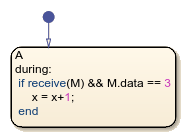Extract message from queue

## Syntax

``receive(message_name)``

## Description

example

````receive(message_name)` extracts an input or local message from its receiving queue. If a valid message exists, `receive` returns `true`. If a valid message does not exist but there is a message in the queue, the chart removes the message from the queue and `receive` returns `true`. If a valid message does not exist and there are no messages in the queue, `receive` returns `false`.```

## Examples

expand all

Check the queue for message `M` and increment the value of `x` if both of these conditions are true:

• A message is present in the queue.

• The data value of the message is equal to 3.

If a message is not present or if the data value is not equal to 3, then the value of `x` does not change. If a message is present, remove it from the queue regardless of the data value.

```during: if receive(M) && M.data == 3 x = x+1; end```## Version History

Introduced in R2015b